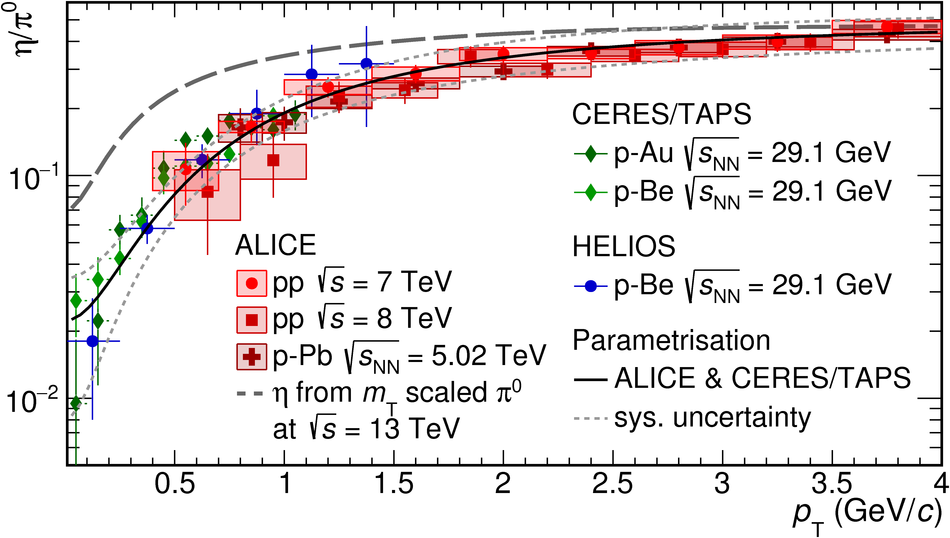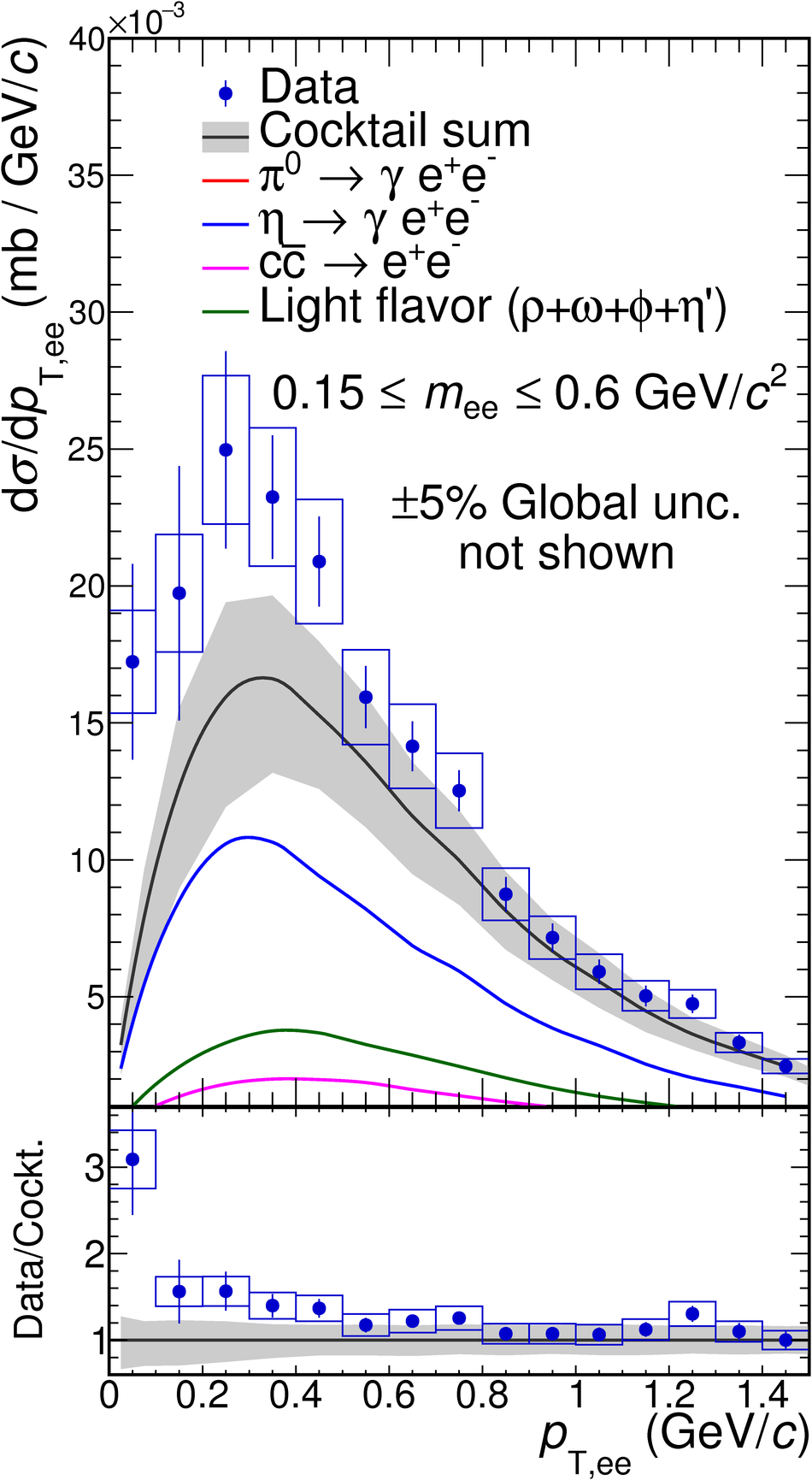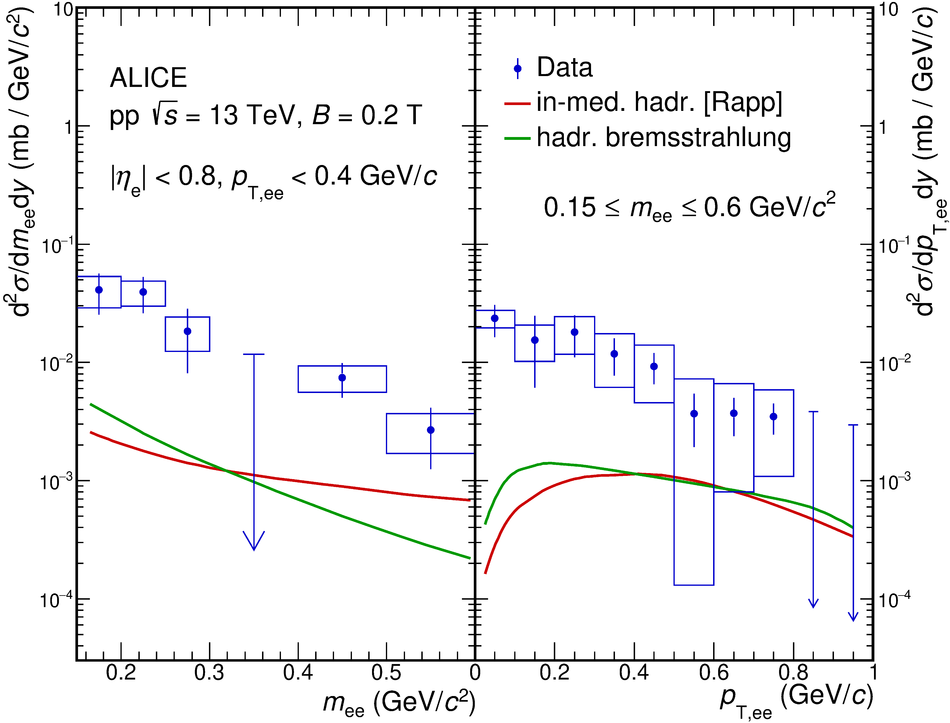# Soft-dielectron excess in proton-proton collisions at $\sqrt{s}$ = 13 TeV

A measurement of dielectron production in proton-proton (pp) collisions at $\sqrt{s} = 13$ TeV, recorded with the ALICE detector at the CERN LHC, is presented in this Letter. The data set was recorded with a reduced magnetic solenoid field. This enables the investigation of a kinematic domain at low dielectron invariant mass $m_{\rm ee}$ and pair transverse momentum $p_{\rm T,ee}$ that was previously inaccessible at the LHC. The cross section for dielectron production is studied as a function of $m_{\rm ee}$, $p_{\rm T,ee}$, and event multiplicity ${\rm d} N_{\rm ch}/{\rm d} \eta$. The expected dielectron rate from hadron decays, called hadronic cocktail, utilizes a parametrization of the measured $\eta/\pi^0$ ratio in pp and proton-nucleus (p-A) collisions, assuming that this ratio shows no strong dependence on collision energy at low transverse momentum. Comparison of the measured dielectron yield to the hadronic cocktail at $0.15<~m_{\rm ee}<~0.6$ GeV/$c^2$ and for $p_{\rm T,ee} <~ 0.4$ GeV/$c$ indicates an enhancement of soft dielectrons, reminiscent of the 'anomalous' soft-photon and -dilepton excess in hadron-hadron collisions reported by several experiments under different experimental conditions. The enhancement factor over the hadronic cocktail amounts to $1.69\pm 0.14\,(\rm{stat.})\pm 0.18\,(\rm{syst., data}) \pm 0.36\,(\rm{syst., cocktail})$ in the ALICE acceptance. Acceptance-corrected excess spectra in $m_{\rm ee}$ and $p_{\rm T,ee}$ are extracted and compared with calculations of dielectron production from hadronic bremsstrahlung and thermal radiation within a hadronic many-body approach.

Submitted to: PRL
e-Print: arXiv:2005.14522 | PDF | inSPIRE

Figures

## Figure 1

 The ratio $\eta/\pi^0$ as a function of \pt, measured in pp and p--A collisions at different center-of-mass energies~. Also shown is the parametrization used for the construction of the hadronic cocktail (solid line), its uncertainty (dotted line), and the expectation from $m_{\rm T}$-scaling (dashed line).## Figure 2

 Differential dielectron cross sections as a function of \mee (left) and \pTee (right). The different components of the hadronic cocktail are shown as solid lines. The error bars and boxes indicate the statistical and systematic uncertainties of the data points. The cocktail uncertainties are shown as gray bands. In the bottom panels, the ratios of data and cocktail are shown.## Figure 3

 Upper panel: Dielectron yield per event in the excess region as a function of the event multiplicity compared with the hadronic cocktail. Lower panel: Enhancement factor data/cocktail in three different kinematic regions. Error bars and boxes show the statistical and systematic uncertainties of the data points. The cocktail uncertainties are indicated as vertical bars around 1 in the lower panel## Figure 4

 Dielectron excess spectra as a function of \mee (left) and \pTee(right) after subtraction of the hadronic decay cocktail. The error bars and boxes represent statistical and combined systematic uncertainties from data and cocktail. Arrows indicate upper limits at 90\% confidence level. Also shown as lines are calculations of bremsstrahlung from initial- and final-state hadrons~, and thermal dielectron production~.